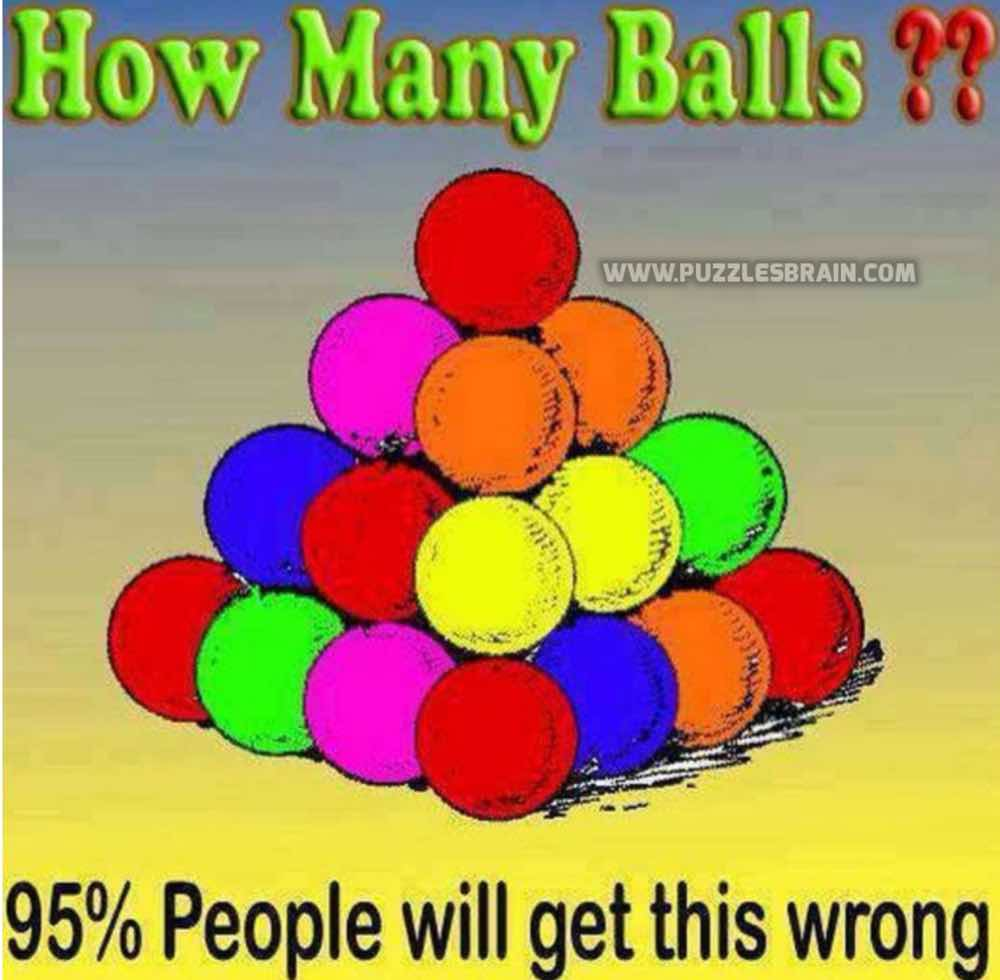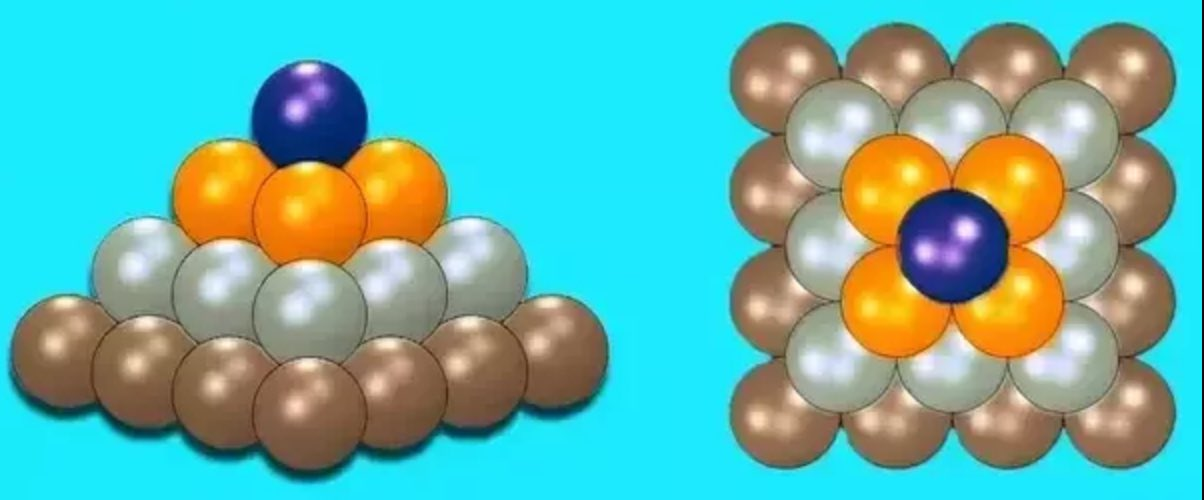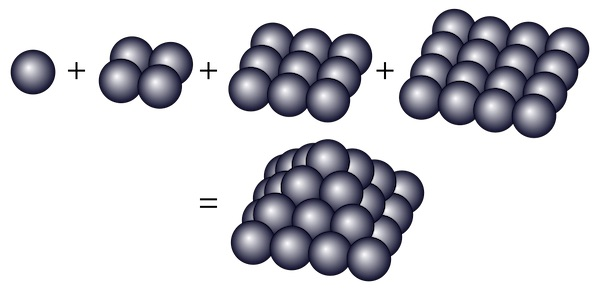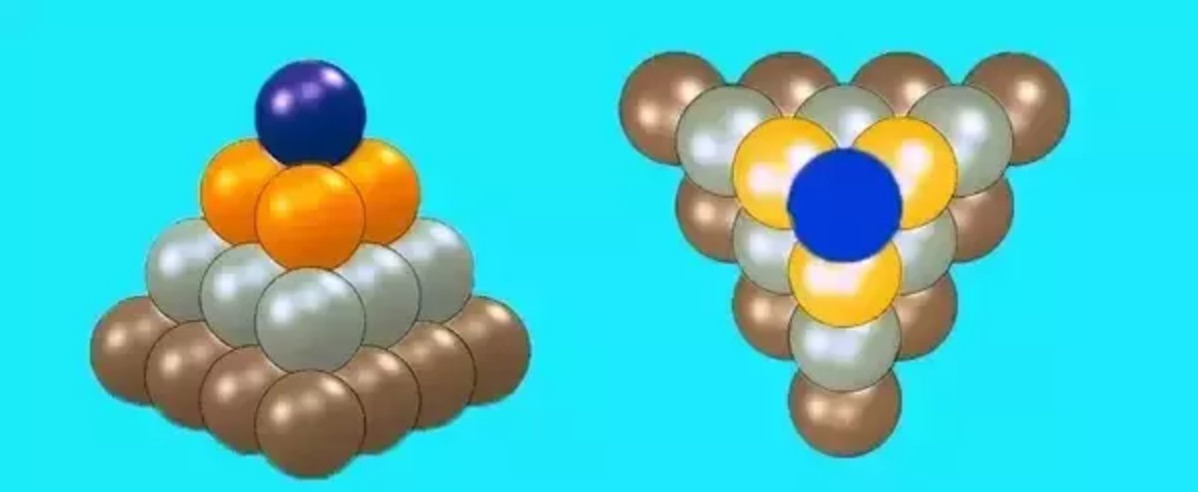# Count number of balls on pyramid puzzleCan you count number of balls on this pyramid?

Many people get it wrong but can you find the correct answer. Try it and then compare your answer with the one given below.

There are 2 possible answers: 30 and 20

If Pyramid is squareIf you remove each layer then you will see this look of each layer:Answer: 16 + 9 + 4 + 1 = 30